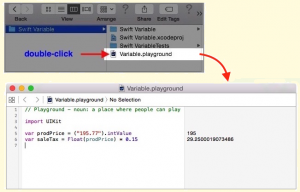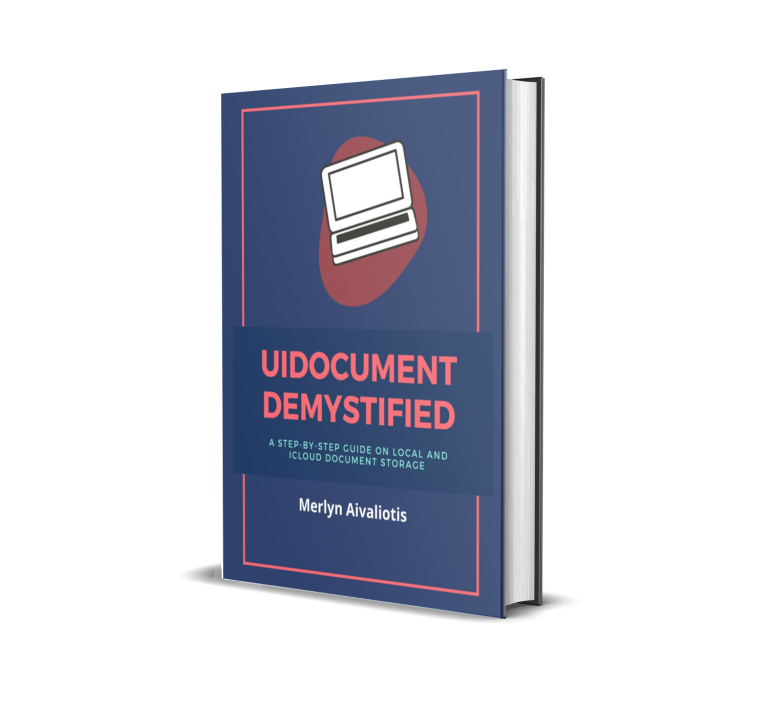Type casting allows you to access a specific value as if it were of a different type. Type casting functions are available in Swift for type casting numbers and strings. In this workshop you learn how to use them. Casting a value using a Swift casting function create a new value that is a direct conversion of the original.

 Before you begin, I assume you’ve done the following: read workshop 1-15 opened the Variable.playground file in Xcode’s IDE-see image below use the playground file to try out code presented in this workshop#### Number Casting Functions [number → number]

This section shows you how to use three functions for casting a number to another type of number.

Double(value) – This function casts the given value as a number (either integer or floating-point).

```var grossPayDouble = Double(17) * 10.15
// Output
172.55
```

Float(value) – this function casts the given value as a number (either integer or double).

```var grossPayFloat: Float = Float(17) * 10.15
// Output
172.549987792969
```

Int(value) – This function casts the given value as a number (either double or floating-point).

```var grossPayInt: Int = Int(17.55) * 10.15
// Output
170
```

#### String Casting Methods [string → number]

Swift provide several convenience methods for type casting a String literal, variable, or constant that have a number to a Float, a Double, or an Int type. This section shows you how to use five of them. All of the convenience methods below skip initial and trailing spaces and characters when they perform the type casting operation.

floatValue – This method typecast a String containing a number to a Float. Code below type cast a string literal, which contain a floating-point number to a Float.

```var prodPrice: Float = ("3737.07").floatValue
// Output
3,737.07006835938```

doubleValue – This method type cast a String to a Double. Code below type cast a String literal which contain a Float to a Double.

```var prodPrice = ("195.77").doubleValue
// Output
195.77```

intValue – This method typecast a String to an Int. Code below type cast a String literal which contain a Float to an Int.

```var aNumber = ("195.77").intValue
var result = aNumber * 4
// Output
195
780```

On line 01 above, the string literal, which contain a floating-point value was type casted to an Int. If we didn’t do that, the compiler would have thrown an error shown below, when we try to use the aNumber variable in a multiplication calculation on line 02.

cannot invoke ‘*’ with an argument list of type ‘(@lvalue String, IntegerLiteralConvertible)’

boolValue – This method type cast a String to a Bool. Here is an example of how to use the boolValue method to type cast a string literal.

```let theLightIsOn = ("Yes").boolValue
if theLightIsOn {
println("Yes, the light IS on")
}
// Output
true
"Yes, the light IS on"```

dataUSingEncoding() This method typecast a String to an NSData.

```let sentence: NSString = "night watch man"
let data = sentence.dataUsingEncoding(NSUTF8StringEncoding)
// Output
"night watch man"
NSConcreteMutableData```

Categories:

Tags:

#### No responses yet

###### UIDocument Demystified: A Step-by-step Guide on Local and iCloud Document Storage